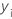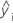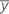Methods and formulas for Best Subsets Regression

Find the method or formula of your choice.

R-sq

R2 is also known as the coefficient of determination.

Notation

TermDescription
yi i th observed response valuemean responsei th fitted response

While the calculations for adjusted R2 can produce negative values, Minitab displays zero for these cases.

Notation

TermDescriptionith observed response valueith fitted responsemean response
nnumber of observations
pnumber of terms in the model

R-sq (pred)

While the calculations for R2(pred) can produce negative values, Minitab displays zero for these cases.

Notation

TermDescription
yi i th observed response valuemean response
n number of observations
ei i th residual
hi i th diagonal element of X(X'X)–1X'
X design matrix

Mallows' Cp

Notation

TermDescription
SSEpsum of squared errors for the model under consideration
MSEmmean square error for the model with all predictors
nnumber of observations
pnumber of terms in the model, including the constant

S

Notation

TermDescription
MSEmean square error
By using this site you agree to the use of cookies for analytics and personalized content.  Read our policy